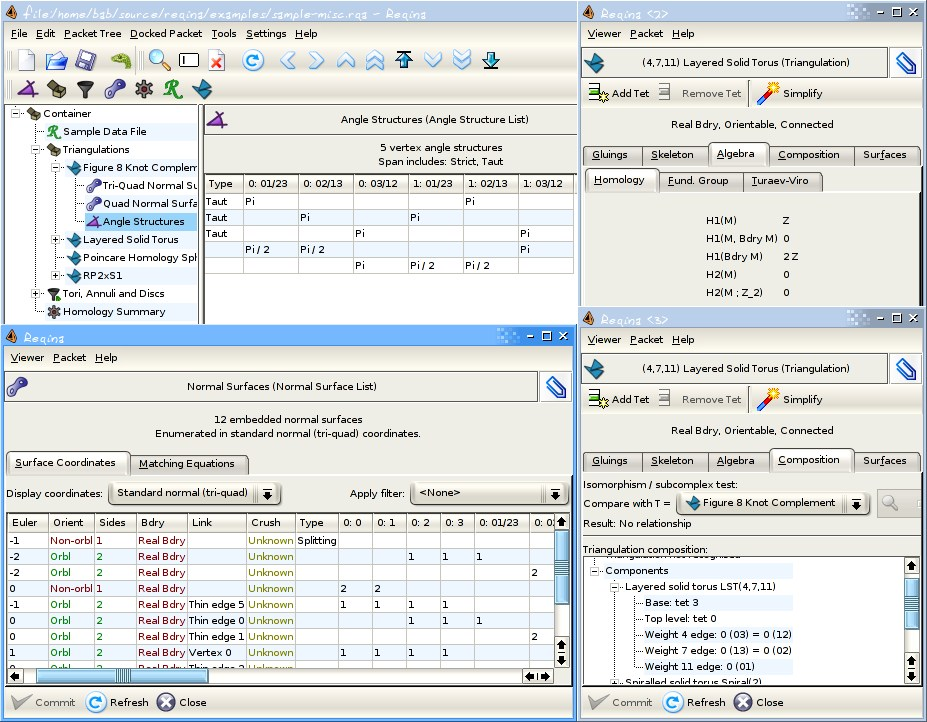## 4 Search Results

### GEOMS

GEOMS is a Fortran77 software package for the numerical integration of general model equations (equations of motion) of multibody systems. The code combines a regularization technique with the Runge-Kutta method of type Radau IIa of order 5. This regularization technique reduces the index under preservation of all information of the solution manifold and, therefore, allows a robust numerical integration of the system.

### polymake

polymake is an object-oriented system for experimental discrete mathematics. The typical working cycle of a polymake user starts with the construction of an object of interest, auch as a convex polytope, a finite simplicial complex, a graph, etc. It is then possible to ask the system for some of the object's properties or for some form of visualization. Further steps might include more elaborate constructions based on previously defined objects. Each class of polymake objects comes with a set of rules which describe how a new property of an object can be derived from previously known ones. It is a key feature that the user can extend or modify the set of rules, add further properties or even new classes of objects (with entirely new rule bases). The functions provided include: several convex hull algorithms, face lattices of convex polytopes, Voronoi diagrams and Delaunay decompositions (in arbitrary dimensions), simplicial homology (with integer coefficients), simplicial cup and cap products, intersection forms of triangulated 4-manifolds. Several forms of (interactive) visualization via interfaces to Geomview, JavaView and other programs.

More information